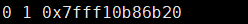1.使用場景

``````#include<stdio.h>
#include <stdlib.h>

int main(int argc, char const *argv[])
{
int a={0,1,2,3,4};
int *b,*d;
int c=2;
int *e=a; //e指向a陣列首地址
//*b=2;   無法直接初始化
//printf("%d\n", *b);
e=e+2;  //移動兩個地址單元
d=&c;  //d指向c的地址來表示值
c=4;   //修改原c變數的值，d指標的值會發生改變
b=(int *)malloc(sizeof(int));//為b分配一個int型空間來直接儲存值
*b=2;//分配空間後可以直接賦值了
printf("this is e,b,c,d :%d %d %d %d\n",*e,*b,c,*d);
return 0;
}``````2.型別說明

（1）int *a :表示一個指向int型變數的指標，指向的是變數的地址單元

（2）char *b:表示一個指向char變數的指標

*a表示的是這個指標指向地址的值，a為此指標本身的地址，這點要明確，一般用*(a+1)、*(a+2)來表示值，如

``````int nums={0,1,2,3,4};
int *a=nums;

printf("%d %d %p\n",*a,*(a+1),a);``````（3）結構體指標

``````typedef struct node{
int data;
struct node * next;

p=(struct node*)malloc(sizeof(struct node)); ``````

p即結構體變數，它本身型別為struc node *型別，使用時，必須為它分配空間或指向此結構體物件地址方能進行下一步操作。

3.指標的運算

（1）值運算：直接通過*運算方式，像a+*(a+1),結果為第一個元素與第二個元素相加。

int nums={0,1,2,3,4};
int *a=nums;

（2）地址運算：通過a+i的方式.指標會指向a的下i個地址。

``````int nums={0,1,2,3,4};
int *a=nums;
a=a+2;
printf("%d\n",*a);``````